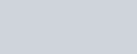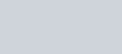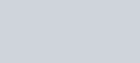Education

# How to solve derivative problems using its rules?Slopes are considered to be an important topic in mathematics, especially in geometry. The slope represents a change of y with regards to x on a straight line. It is the same at every point of a line. But, certainly, this is not the case with a curve line.

So, how do you find the slope of a curve line? It is found by calculating the slope of the secant line passing through two points of the curve.

Above is a graph of the function of x2. We drew a secant line to find the slope between point (1, 1) and point (2, 4),

But what if we wanted to find the slope of a point at the exact point (1,1), the instantaneous rate of change?

# What exactly is a derivative?

Derivatives are used to find the slope of a curve line at an exact point. Derivatives can be defined as:

The derivative of a function can be interpreted as the slope of the graph of the function or, more precisely, as the slope of the tangent line at a point.

In calculating derivatives, we find the differential of a function. Meaning here that, we find the difference between the two points.

These points are placed so closely together that the difference between them is almost zero and the secant line between them becomes the tangent line.

Derivatives are notated in three different ways. The most common way is Labinez’s notation.

# How to perform differentiation?

Hopefully, now your confusion about derivatives and why we use them is clear. Let’s move on to the calculation of derivatives.

There are two main ways by which derivatives are solved. One is formal and the other is an alternate form. We will learn both with general examples. However, derivatives can be dealt with using a derivative calculator.

## 1.   Explicit Differentiation

It will be easy to derive a formula for derivatives while looking at a graph.

Consider a sinusoidal function. We want to calculate the slope between two points on this curve. The first point is at x. As we know y=f(x), we can write the point as (x,f(x)).

We will take the second point at a distance of h from x. The point here will be (x+h,f(x+h)), where h is an arbitrary value. It could be any number.

Slope at this point is:As we want to calculate the slope of the exact point, we will bring the two points so close together that they almost become one point and the difference between them approaches zero.

For this purpose, we will apply a limit on this function. The equation becomes like this:This is what we will call derivative or d over dx (d/dx). Now, we can substitute any value at the place of x.

So this was one way to calculate the derivative or find the slope at a pinpoint. Let’s move on to the second and alternative formula of differentiation.

Usually in calculus,  replaces h. Delta means a very small change. The slope formula with Δχ is:This is quite similar to the first formula. But here instead of adding a distance to x, we will take a whole different point (a,f(a)).

On applying the slope formula, the equation will be:On applying limit:This is the same thing as the formal way. Here, instead of taking a distance h, we took a direct point a. So when x approaches a the distance will be almost zero. And this is exactly what we want to calculate.

## Example:

Find the derivative of f(x) = x2.

## Solution:

Step 1: Add the distance of in the x2. This distance of delta x is approximately zero. We will now find the function for (x + Δχ)2.

= (x + Δχ)2

f(x + Δχ)  = x2 + 2(x)(Δχ) + (Δχ)2

Step 2: Applying slope formula is:Step 3: Apply limit approaches to zero.Hence, the slope at x2 = 2x.

You can verify the result by using an online derivative calculator.

## 2. Implicit Differentiation

Since you now have an idea about solving derivatives. It is about the right time we see how to perform implicit differentiation without using an implicit differentiation calculator.

Implicit equation is an equation in which variables cannot be separated.

Implicit differentiation is simple differentiation except that it is performed on both sides of the equation.

Example

Differentiate this implicit equation:

x2 + y2 = z2

Where z is constant.

## Solution:

Step 1: Apply derivative.

d/dx (x2) + d/dx(y2) = d/dx(z2)

Step 2: Solve left hand side.

= d/dx (x2) + d/dx(y2)

= 2x + 2y(d/dx)

Step 3: Solve right hand side.

The derivative of constant numbers is 0.

= d/dx (z2)

= 0

Step 4: put the values in the equation.

2x + 2y(d/dx) = 0

2y(d/dx) = -2x

y(d/dx) = -x

d/dx = -x/y

By differentiating the equation x2 + y2 = z2, we got -x/y as derivative. To reverse this derivation, an antiderivative calculator could be a handy tool to take the antiderivative of this function.

### Mursaleen

Hi. I'm Mursaleen Siddique, The guy behind UltraUpdates.com. I'd rather call myself a struggling Blogger. I love Blogging with WordPress, Covering Tech, General Topics, Graphic & Web Design Inspiration., Feel free to get in touch via mentioned social media platform or E-mail me at hello[at]ultraupdates.com

### Related Articles

This site uses Akismet to reduce spam. Learn how your comment data is processed.